# Some Applications of Trigonometry Class 10th Solutions

Line Of Sight: An Imaginary line drawn between the object and the eyes of the observer.

The angle of elevation of the point viewed by the observer is made by the line of sight with above the horizontal level.

note: In this situation, we always raise our heads to watch the object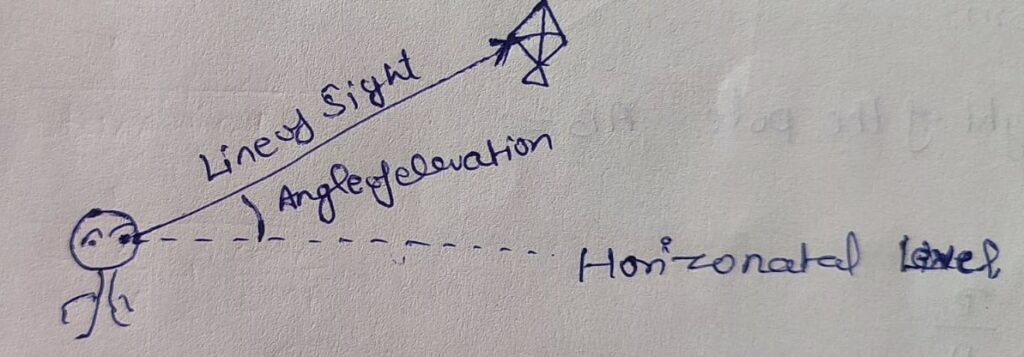Angle Of Depression:    The angle of depression of an object is the angle made by the line of sight and below the horizontal level.

note: In this situation, We always down our heads to look at the object.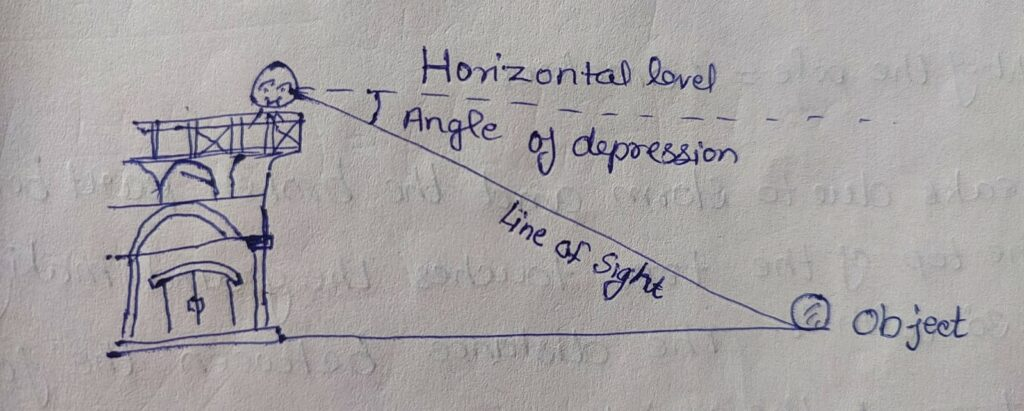## Exercise 9.1

Q1. A circus artist climbing a 20m long rope, which is tightly stretched and tied from the top of a vertical pole to the ground. Find the height of the pole, if the angle made by the rope with the ground level is 30º

Given: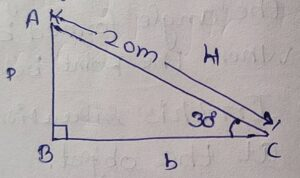In Δ ABC

∠B = 90º

θ = 30º

AC = 20m

To Find:

Height of the pole AB =?

Solution:

AB/AC = P/H

=> AB/20 = sinθ

=> AB = 20sin30º

=> AB = 20×1/2                     { sin30º=1/2  }

=> AB = 10m

Hence Height of the pole = 10meters

Q2. A tree breaks due to a storm and the broken part bends so that the top of the tree touches the ground making an angle 30º with it. The distance between the foot of the tree to the point where the top touches the ground is 8m. Find the height of the tree.

Given: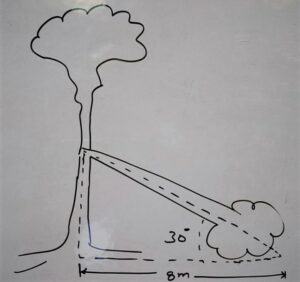According to figure

In Triangle ABCangle of elevation θ = 30º

BC = 8m

To Find:

Height of the tree (AB+AC) =?

Solution:

In triangle ABC

∠B = 90º

BC = 8m

AC = ?

AB =?

AC/BC = hypotenuse(H)/Base(b)

=> AC/8 = secθ

=> AC/8 = sec 30º

=> AC = 8 sec 30º

=> AC = 8 × 2/√3

=> AC = 16/√3      ………………equation (i)

now for AB = ?

AB/BC =perpendicular(p)/Base(b)

=> AB /8 = tanθ

=> AB/8 = tan 30º

=> AB = 8 × 1/√3

=> AB = 8/√3         ………………………..equation (ii)

AB+ AC = 8/√3 + 16/√3

=> AB + AC = 24/√3

=> AB + AC = 24/√3  ×√3 /√3

=> AB + AC = 24√3/3

=> AB + AC = 8√3

Hence Height of tree =  8√3 m

Q3. A contractor plants to instant two slides for the children to play in a park. For children below the age of 5 years, she prefers to have a slide whose top is at a height of 1.5m and is inclined at an angle of 30º to the ground, whereas for elder children she wants to have a steep slide at an angle if 60º to the ground what should be the length of the slide in each case?

Given:

Case Ist: Below the age of 5 years children

In ΔABC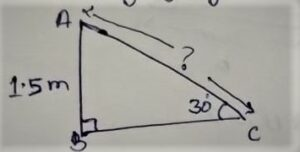AB=1.5m

θ=30º

Ac=?

In Case IInd: For 5 years elder children

In ΔPQR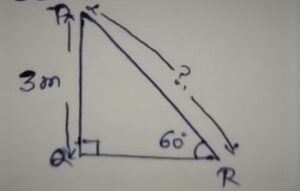PQ= 3m

θ=60º

PR=?

Solution:

For case Ist:

AC/AB = H/P

=>AC/1.5 = Cosecθ

=>AC/1.5 =cosec30º

=>AC = 1.5×2

=>AC= 3.0

=>AC=3m

For Case IInd:

PR/PQ = H/P

=>PR/3 = cosecθ

=>PR/3 = cosec60º

=>PR/3 = 2/√3

=>PR = 6/√3  ×√3 /√3

=>PR=6√3 /(√3 )²        {After rationalising the denominator}

=>PR = 6√3 /3

=>PR=2√3 m

Hence

• Length of the slide for the age of 5 years = 3 meters
• Length of the slide for the elder age of 5 years = 2√3  meters

Q4. The angle of elevation of the top of a tower From a point om the ground, which is 30m away from the foot of the tower, is 30°. Find the height of the tower.

Given: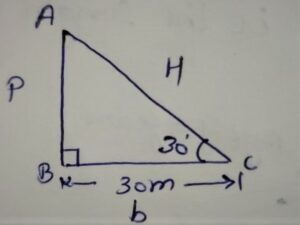In ΔABC

BC=30m

θ=30º

To Find:

Height of the tower AB = ?

Solution:

In ΔABC

AB/BC = P/b

=>AB/30 = tanθ

=> AB/30 = tan30º

=>AB/30 = 1/√3

=>AB = 30×1/√3

=>AB=30×1/√3    ×  √3 /√3  {After Rationalise the denominator }

=>AB=30√3 /(√3 )²

=>AB=30√3 /3

=>AB=10√3 m

Hence height of the tower 10√3  meters

Q5. A kite is Flying at a height of 60m above the ground. The string attached to the kite is temporarily tied to a point on the ground is 60º. Find the length of the string. Assuming that there is no slack in the string.

Given: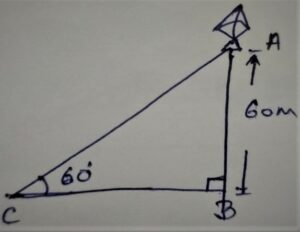In ΔABC

θ=60°

AB=60m

To Find:

The length of the string AC=?

Solution:

In ΔABC

AC/AB=H/P

=>AC/60=Cosecθ

=>AC/60=Cosec60°

=>AC=60× 2/√3

=>AC=120/√3  × √3/√3 { Rationalise the denominator }

=>AC=120√3/(√3)²

=>AC=120√3/3

=>AC=40√3

Hence the length of the string AC= 40√3 meters

Q6. A 1.5m tall boy is standing at some distance From a 30m tall building. The angle of elevation From his eyes to the top of the building increases From 30º to 60° as he walks towards the building. Find the distance he walked towards the building.

Solution:

According to the figure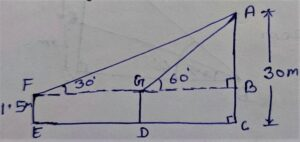EF=BC=1.5m

Height of building(AC)=30m

AB=AC-BC

=>AB=30-1.5

=>AB=28.5m

In ΔABG

∠B=90°

∠G=θ=60°

AB=28.5m

BG=?

BG/AB = b/P

=>BG/AB = Cotθ

=>BG/28.5 = Cot60°

=>BG/28.5 = 1/√3

=>BG=28.5/√3  × √3/√3   { Rationalize the denominator }

=>BG=28.5√3/(√3)²

=>BG=28.5√3/3

=>BG=9.5√3      ………………….(i)

In ΔABF

∠B = 90°

∠F = θ = 30°

BF =?

BF/AB = b/P

=>BF/AB = Cotθ

=> BF/28.5 = Cot 30°

=>BF /28.5 = √3

=> BF = 28.5√3    ………………….(ii)

FG= BF – BG

=> FG = 28.5√3 – 9.5√3

=>FG = 19.0√3

=>FG = 19√3

Hence The distance he walked towards the building =  19√3 meters

Q7.From a point on the ground, the angles of elevation of the bottom and the top of a transmission tower Fixed at the top of 20m high building are 45° and 60º respectively. Find the height of the tower.

Given: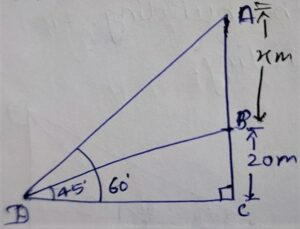Height of building BC=20m

To Find:

Height of tower=?

Solution:

According to figure

Let the Height of the tower be = x meters

Now in ΔBCD

∠C = 90º

∠BDC=θ =45º

CD =?

CD/BC = b/P = Cotθ

=> CD/ 20 = Cot45º

=> CD/20 = 1

=>CD = 20         ……………………(i)

In ΔACD

AC=AB+BC

=>AC= x+20

θ=60º

DC=?

DC/AC = b/P

=>DC/x+20 = Cotθ

=>DC/x+20 = Cot60°

=>DC = x+20 ×1/√3

=>DC = x+20 / √3       …………..(ii)

Equation(i) = Equation(ii)

CD=DC

=>20=x+20/√3

=>20√3 = x+20

=>20√3-20 = x

=>20(√3-1) =x

Hence Height of the tower = 20(√3-1)meters

Q8. A Statue,1.6m tall, stands on the top of a pedestal. From a point on the ground, the angle of elevation of the top of the statue is 60º and From the same point, the angle of elevation of the top of the pedestal is 45º. Find the height of the pedestal.

Given:

According to figure

Height of statue AB=1.6m

∠BDC = 45º

To Find:

Height of Pedestal BC= x (Let)

Solution:

In ΔACD

AC=AB+BC

=>AC= 1.6+X

θ=60º

DC=?

DC/AC=b/p

=>DC/1.6+x  = Cotθ

=>DC/1.6+x = Cot60°

=>DC/1.6+x = 1/√3

=> DC = 1.6+x /√3           ……………………….(i)

In ΔBCD

BC=x

θ=45º

DC=?

DC/BC = b/P

=>DC/x = Cotθ

=>DC/x=Cot45º

=>DC/x = 1

=>DC =x         …………………………(ii)

Equation (i) = Equation(ii)

DC=DC

=>1.6+x /√3 = x

=>1.6+x =√3 x

=> 1.6 = √3 x – x

=>1.6 = (√3  – 1)x

=>1.6/ (√3  – 1)  = x

=>1.6/ (√3  – 1)  × (√3  + 1)/ (√3  + 1) = x  Rationalize the denominator }

=>1.6 (√3  + 1))/(3-1)= x

=>1.6 (√3  + 1)/2 =x

=>0.8 (√3  + 1)=x

Hence Height of the Pedestal = 0.8( (√3  + 1)meters

Q9. The angle of elevation of the top of a building From the foot of the tower is 30º and the angle of elevation of the top of the tower from the foot of the building is 60°. If the tower is 50m high, Find the height of the building.

Given:

According to figure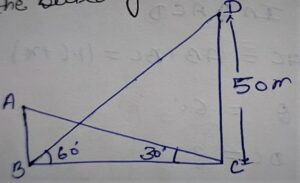Height of tower CD = 50m.

∠ACB = 30º

∠CBD = 60º

To Find:

Height of the building (AB)=?

Solution:

AB=Let  the Height of the building be = x meters

In ΔDCB

θ=60°

DC = 50 m

BC= ?

BC/DC =b/P

=> BC/50 = Cotθ

=>BC/50 = Cot60º

=>BC/50 = 1/√3

=>BC = 50/√3                ……………………(i)

In ΔABC

AB= x (Let)

θ= 30°

BC=?

BC/AB = b/P

=>BC/x = Cotθ

=>BC/x = Cot30º

=>BC/x = √3

=>BC= √3 x      …………………(ii)

Equation(i)=Equation(ii)

50/√3 = √3x

=>50/√3 = √3x

=> 50 =(√3)²x

=>50 = 3x

=> 50/3 = x

=> 16.66m

Hence the Height of the building is 16.666m

Q10. Two poles of equal height are standing opposite each other on either side of the road, which is 80m wide. From a point between them on the road, the angles of elevation of the top of the pole are 60º and 30° respectively. Find the height of the poles and the distances of the point From the poles.

Given:

According to figure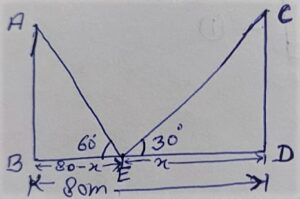AB and CD are poles

BD= Distance between two poles =80m

∠AEB= 60º

∠CED = 30º

To Find:

(i)Height of poles = AB = CD = ?

(ii)Distances of the points E From the poles=?

Solution:

In ΔCDE

ED = x (Let)

θ = 30º

CD =?

CD/ED = P/b

=> CD/ED = tanθ

=> CD/x = tan 30º

=> CD = x tan 30º

=> CD = x × 1/√3

=> CD = x/√3  ……..(i)

Similarly In Δ AEB

θ = 60º

BE = (80 – x)meter

AB =?

AB/BE = P/b

=> AB/(80-x) = tan θ

=> AB/80 – x = tan 60º

=> AB = (80 – x)√3 …..(ii)

We know that Height of both poles are equal

Equation (i) = Equation (ii)

CD = AB

=> x/√3 =(80 – x) √3

=> x = (80 – x)√3×√3

=> x = (80 – x)3

=> x = 240 – 3x

=> x + 3x = 240

=> 4x = 240

=> x = 240/4

=> x = 60

=> x = 60 put into Equation (ii)

AB = (80 – 60) √3

AB = 20 √3

Hence:

(i) Height of each pole = 20√3

(ii) Distances of point E From the poles are 60meters and 20 meters.

Q11. A TV tower stands vertically on a bank of a canal . From a point on the other bank directly opposite the tower, the angle of elevation of the top of the tower is 60º. From another point, 20m away From this point o the line joining the point to the foot of the tower, the angle of the top of the tower is 30º(see in figure) Find the height of the tower and the width of the canal.

Solution:

According to figure: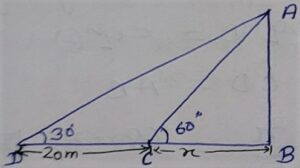AB = TV tower

CB = width of the canal.

∠ACB = 60º

To Find:

(i) Height of the tower (AB) =?

(ii) Width of the canal CB =?

Solution:

In Δ ABC

θ = 60º

BC = x (Let )

AB = ?

AB/BC = P/b

=> AB/x = tan θ

=> AB/x = tan 60º

=> AB = √3 x …..(i)

In Δ ABD

BD = BC + CD

=>BD= (x + 20)

θ = 30º

AB =?

AB/BD = p/b

=> AB/BD = tan θ

=> AB/x+20 = tan 30º

=> AB = (x+20)×1/√3

=> AB = (x+20)/√3 …..(i)

Now Equation (i) = Equation(ii)

AB = AB

=> √3x = (x+20)/√3

=> 3x = (x+20)

=> 3x – x = 20

=> 2x =20

=> x = 20/2

=> x = 10 Put into equation (i)

AB = √3×10

=> AB = 10√3 meters

Hence: (i) Height of tower AB = 10√3m

(ii)Width of the canal CB = 10m

Q12. From the top of a 7m high building, the angle of elevation of the top of a cable tower is 60º and the angle of depression of its foot is 45°. Determine the height of the tower.

Given:

According to figure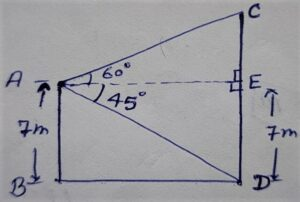Height of the building AB = 7m

∠CAE = 60º

CD = cable tower

To Find:

Height of the tower CD =?

Solution:

In Δ AED

∠AED = 90º

Here AB = ED = 7m

AE = BD =?

AE/ED = b/p

=> AE/7 = cot θ

=> AE/7 = cot 45º

=> AE = 7 × 1

=> AE = 7

Now In Δ CEA

AE = 7

θ = 60º

CE = ?

CE/AE = P/b

=> CE/7 = tan θ

=> CE/7 = tan 60º

=> CE/7 = √3

=> CE = 7√3

Hence:

Height of tower CD = CE + ED

=> Height of tower CD = 7√3 + 7

=> Height of tower CD = 7(√3 + 1)m

Q13. As observed from the top of a 75 m lighthouse from the sea level, the angles of depression of two ships are 30º and 45º. If one ship is exactly behind the other on the same side of the lighthouse, find the distance between the two ships.

Given:

According to figure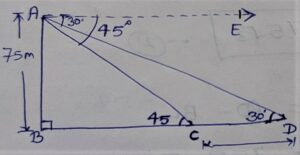AB = Height of lighthouse = 75 m

∠EAC = 45º

To Find:

Distance between two ships = CD =?

Solution:

In Δ ABC

AB = 75 m

∠EAC = ∠ACB = θ = 45º {Alternate interior angle}

BC =?

BC/AB = b/p

=> BC/AB = cotθ

=> BC/75 = cot 45º

=> BC = 75 × 1

=> BC = 75 m ……….(i)

In Δ ABD

AB = 75 m

BD =?

BD/75 = cot 30º

=> BD = 75√3  ………..(ii)

CD = BD – BC

=> CD = 75√3  – 75

=> CD = 75(√3 – 1)

Hence Distance between two ships = 75(√3 – 1) m

Q. 14.  A 1.2 m tall girl spots a balloon moving with the wind in a horizontal line at a height of 88.2 m from the ground. The balloon’s angle of elevation from the girl’s eyes at any instant is 60º. After some time, the angle of elevation reduces to 30º. Find the distance travelled by the balloon during the interval.

Given:

According to figure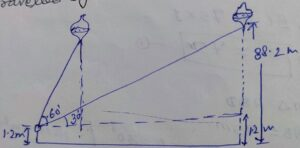AC = 88.2 m

ED = BC = 1.2 m

To Find:

Distance Travelled by the balloon FB =?

Solution:

According to figure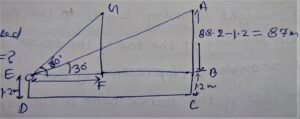In Δ ABC

AB = AC – BC

=> AB = 88.2 – 1.2

=> AB = 87 m

θ = 30º

BE =?

BE/AB = b/p

=> BE/87 = cot θ

=> BE/87 = cot 30º

=> BE = 87 × √3

=> BE = 87√3  ………(i)

In Δ GFE

θ = 60º

GE = AB = 87

FE =?

FE/AB = b/P

=> FE/AB = cot θ

=> FE/AB = cot 60º

=> FE/87 = 1/√3

=> FE = 87 × 1/√3

=> FE = 87/√3     ………(ii)

FB = EB – EF

=> FB = 87√3 – 87/√3   { From equation (i) and equation (ii) }

=> FB = (87×3 – 87) /√3 { taking √3 as LCM }

=> FB = (261 – 87)√3

=> FB = 174/√3 × √3/√3

=> FB = 174√3/3

=> FB = 58√3 m

Hence the Distance travelled by the balloon = 58√3 m.

Q.15. A straight highway leads to the foot of a tower. A man standing at the top of the tower observes a car at an angle of depression of 30°, which is approaching the foot of the tower with a uniform speed. Six seconds later, the angle of depression of the car is found to be 60º. Find the time taken by the car to reach the foot of the tower from this point.

Given: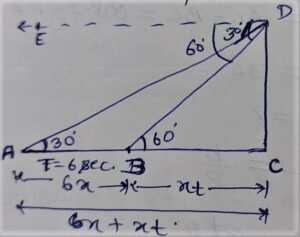According to figure

DC = tower

∠EDA = ∠DAC = 30º

∠EDB = ∠DBC = 60º

To Find :

Time is taken by car to reach the foot (c) of the tower From point B =?

Solution:

According to figure:

Let the uniform speed of the car be = x m/sec.

AB = Distance covered by car in 6 seconds =speed × time

=> AB = Distance covered by car in 6 seconds  = x × 6=6x m

Let time taken by car from B to C be = t seconds

∴ Distance BC = speed × time =

=>Distance BC = x × t =x t

In triangle BCD

∠DBC = ∠EDB =θ =60º

DC =?

DC/BC = perpendicular(p)/base(b)

=> DC/x t = tanθ

=>DC/x t = tan60º

=> DC = √3×xt

In Δ ACD

AC = AB + BC =6x +xt

θ =30º

DC =?

DC/AC = Perpendicular(p)/Base(b)

=> DC/AC = tanθ

=>DC/6x+xt = tan30º

=> DC/6x+xt =1/√3

=> DC = (6x+xt)×1/√3

=> DC = (6x+xt)/√3  ……………..equation(ii)

Equation(i) = Equation(ii)

√3 xt = (6x+xt)/√3

=> √3 x t ×√3 = (6x+xt)

=> 3 x t = 6x + x t

=> 3xt – x t = 6x

=> 2xt = 6x

=> t =6x/2x

=> t =3 seconds

Hence time taken by car to reach the foot of tower from point B = 3seconds

Q16. The angle of elevation of the top of a tower From two points at a distance of 4m and 9m.From the base of the tower and in the same straight line with it are complementary .Prove that the height of the tower is 6m.

Given: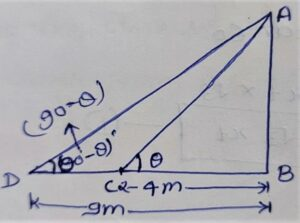According to figure

AB = Height of tower

BC = 4m

BD = 9m

To Prove:

Height of tower AB = 6m

Solution:

In triangle ABC

BC = 4m

∠ACB = θ

AB = ?

AB/BC = perpendicular(p)/base(b)

=> AB/4 = tanθ

=> AB = 4 tanθ …………………………….equation(i)

In triangle ABD

BD = 9m

AB =?

AB/BD = perpendicular(p)/base(b)

AB/9 = tan(90º-θ)

=> AB = 9 cotθ  {by Applying tan(90º-θ) = cotθ } ……………………..equation(ii)

equation(i) × equation(ii)

AB×AB = 4 tanθ × 9 cotθ

=> (AB)² = 4×9 × tanθ ×  cotθ

=> (AB)² = 4×9 × tanθ ×  1/tanθ  { by applying cotθ = 1/tanθ }

=> (AB)² = 36

=> AB = √36

=> AB = 6

Hence Height of tower = 6m

Hence proved